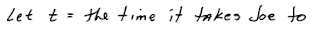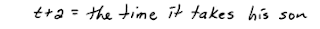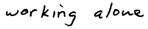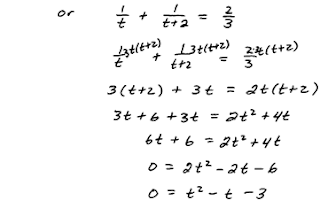## Pages

### Guidelines for Solving Quadratic Equations and ApplicationsThe argument of the square root, b^2 - 4ac, is called the discriminant and can be used to determine the number and type of solutions to the quadratic equation without doing all the work to find the actual solutions.Furthermore, if the discriminant is negative we will obtain two nonreal solutions (complex solutions).  If the discriminant is a perfect square, then the equation will have rational solutions.

Use the Discriminant to determine the number and type the solutions.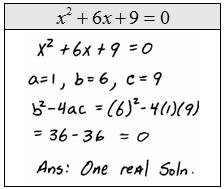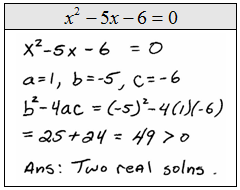If the discriminant is a perfect square then the quadratic was factorable. In this case, it is usually more efficient to solve by factoring and should be done that way unless stated otherwise.  However, notice that whichever method you choose you should obtain the same answers.

Solve.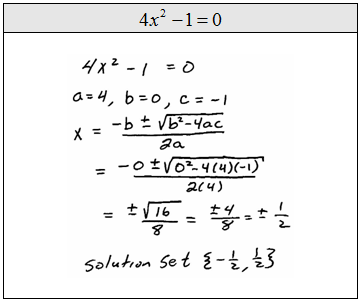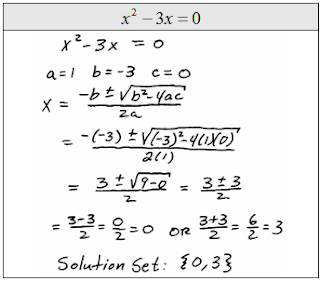The word problems in this chapter should seem familiar. The set-ups are similar but usually lead to quadratic equations.  You could use the quadratic formula or any one of the other techniques learned so far to solve them.

Geometry Problem: The area of a square is 32 square feet. Find the length of each side of the square.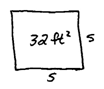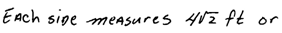Geometry Problem: The area of a circle is  square centimeters. Find the length of the diameter.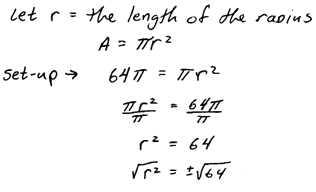Geometry Problem: The length of a rectangle is 2 less than 3 times its width. If the area measures 65 square meters find the dimensions of the rectangle.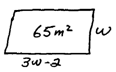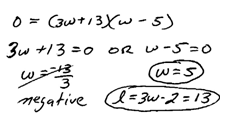Tip: Be sure to include the proper units in your answer. Most instructors will mark off a few points if you neglect the units.

Geometry Problem: The base of a triangle measures twice that of the height. If the area of the triangle is 25 square inches find the length of the base and height.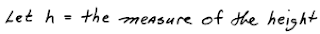For any right triangle the sum of the squares of the legs will equal the square of the hypotenuse. Use this relationship  to find any missing part of a triangle given any two of the other sides.

Pythagorean Theorem Problem: The lengths of the legs of a right triangle are 6 inches and 8 inches. Find the length of the hypotenuse.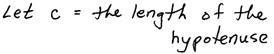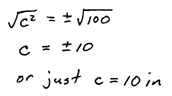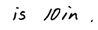Pythagorean Theorem Problem: The base of a 10 foot ladder is positioned 4 feet from a wall and leaned against it. Determine how high the ladder reaches.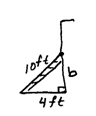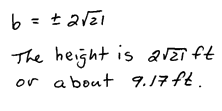Pythagorean Theorem Problem: A computer screen has dimensions 14.5 inches by 11 inches. Find the length of the diagonal.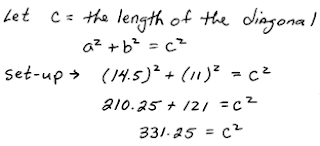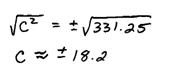Pythagorean Theorem Problem: If Joe traveled 100 miles south and 50 miles west to the beach, then how far from home is he?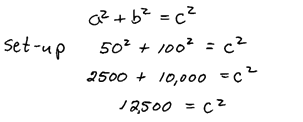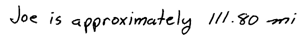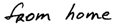If an object is launched from a height of s feet with an initial speed of  feet per second, its height is given by the following formula.Projectile Problem
: An object is thrown from the ground at an initial speed of 32 feet per second. How will it take for the object to come back to the ground?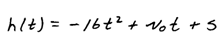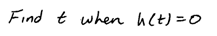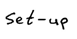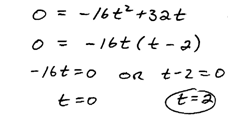Projectile Problem: An object is launched from the top of a 32 foot building at an initial speed of 128 feet per second. How long will it take to reach the ground?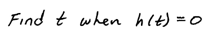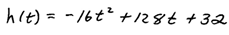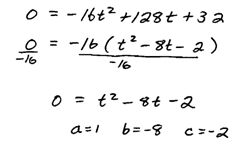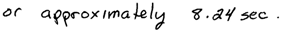Projectile Problem: An object is launched from ground level at a speed of 128 feet per second. How long will it take to reach a height of 256 feet?Recall the work-rate formulas from Chapter 7.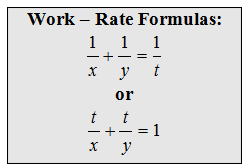Work-Rate Problem: It usually takes Joe’s son 2 hours more time working alone to do the weekly yard work. If Joe and his son do the work together it takes 1 1/2 hours. How long would it take Joe to do the yard working alone?